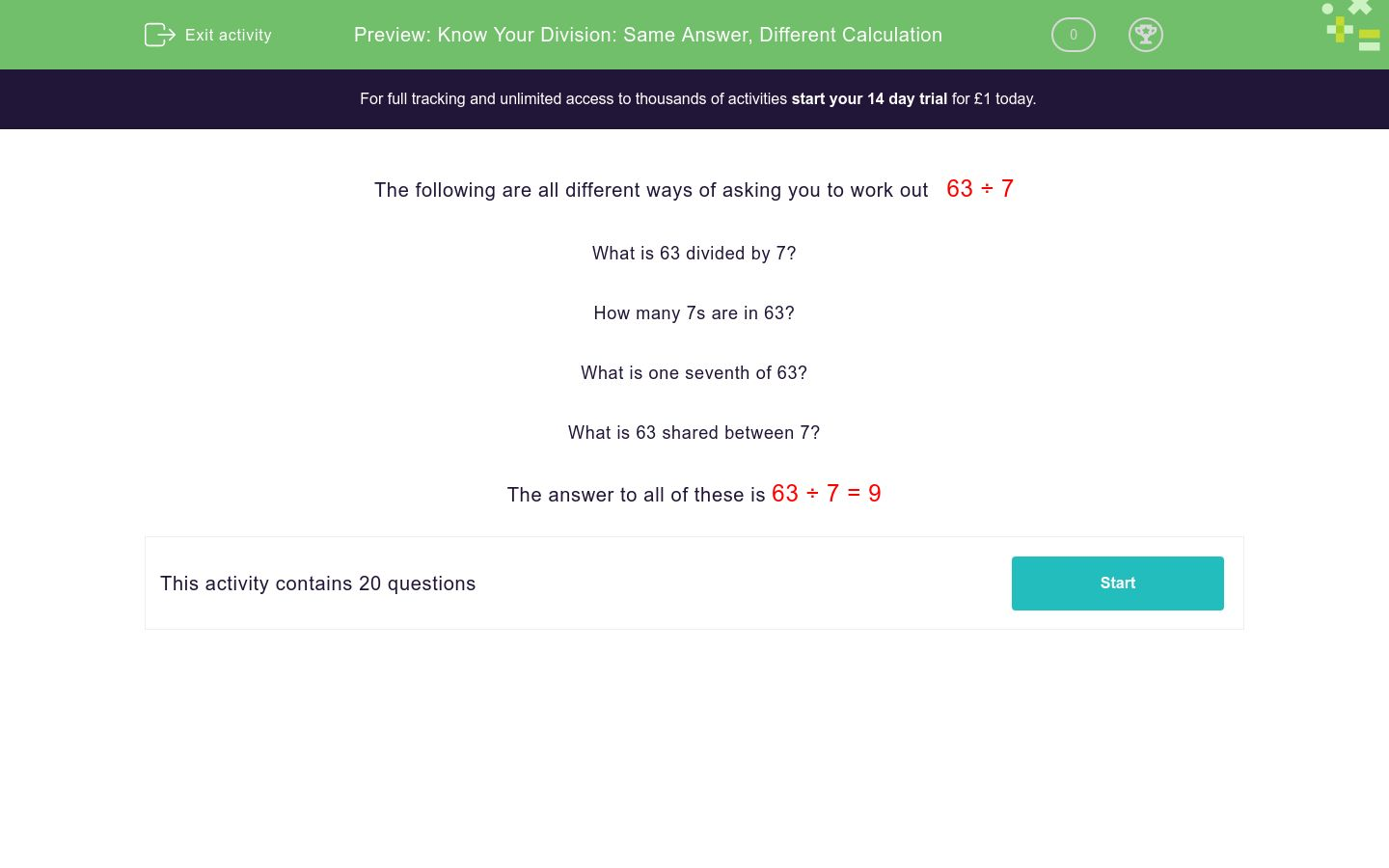### Comprehensive & curriculum aligned

In this worksheet, students answer quick division questions set out in a variety of ways.Key stage:  KS 2

Curriculum topic:   Number: Multiplication and Division

Curriculum subtopic:   Recognise/Use Pairs in Mental Calculation

Difficulty level:### QUESTION 1 of 10

The following are all different ways of asking you to work out   63 ÷ 7

What is 63 divided by 7?

How many 7s are in 63?

What is one seventh of 63?

What is 63 shared between 7?

The answer to all of these is 63 ÷ 7 = 9

---- OR ----